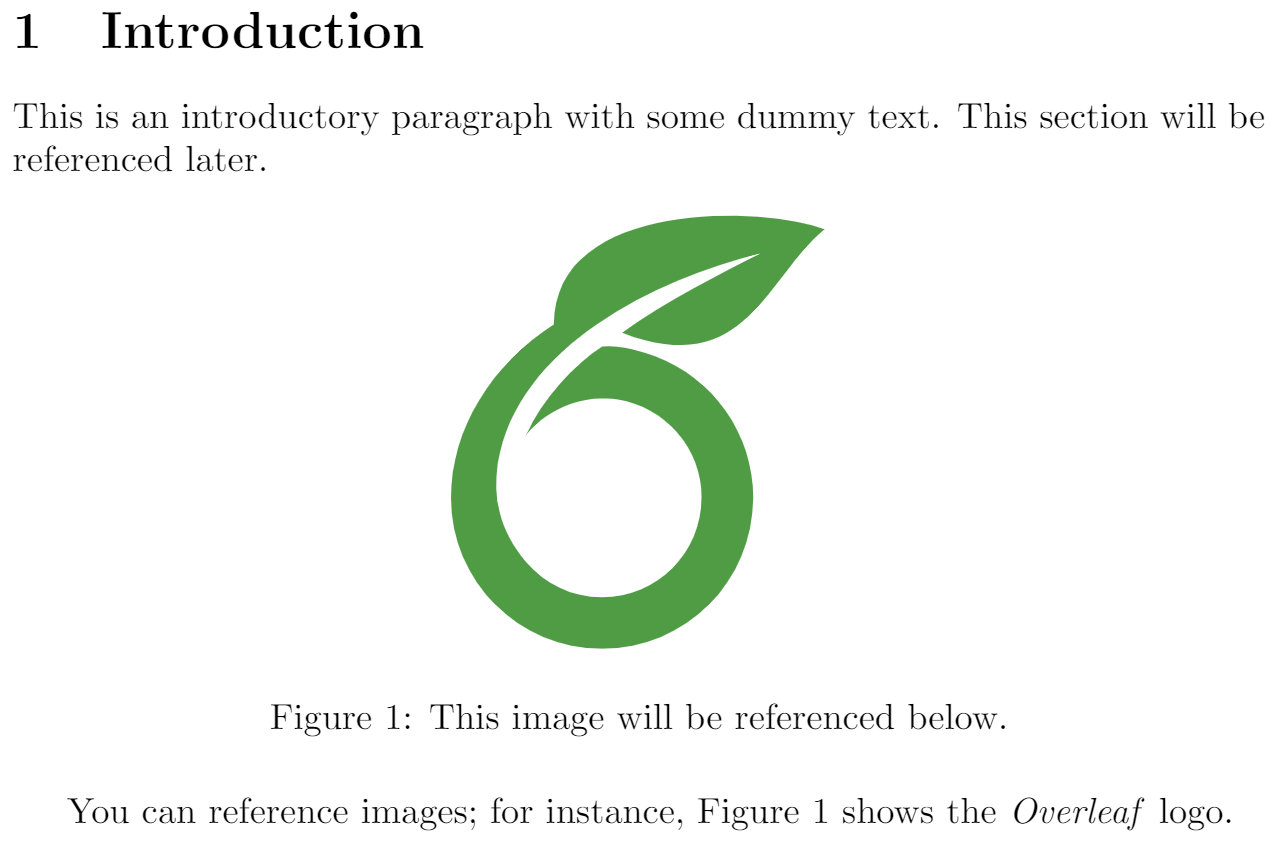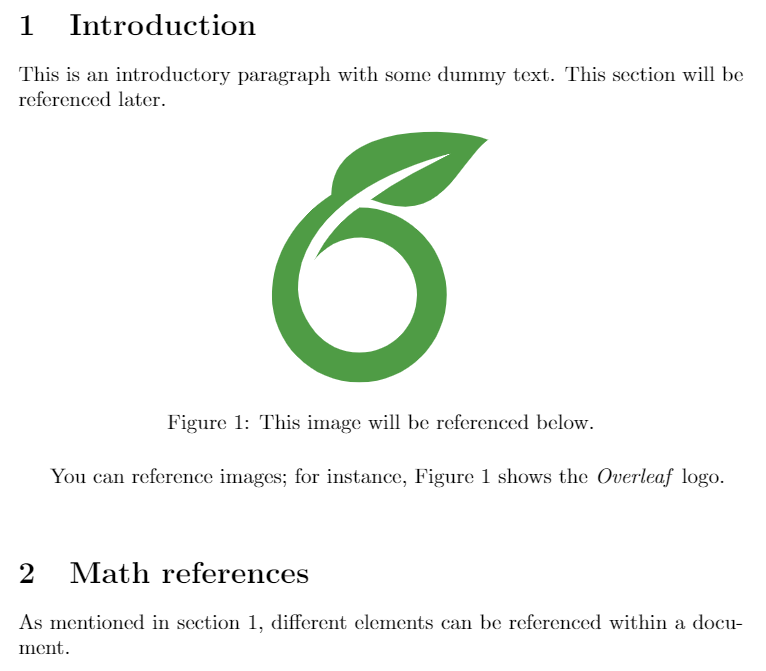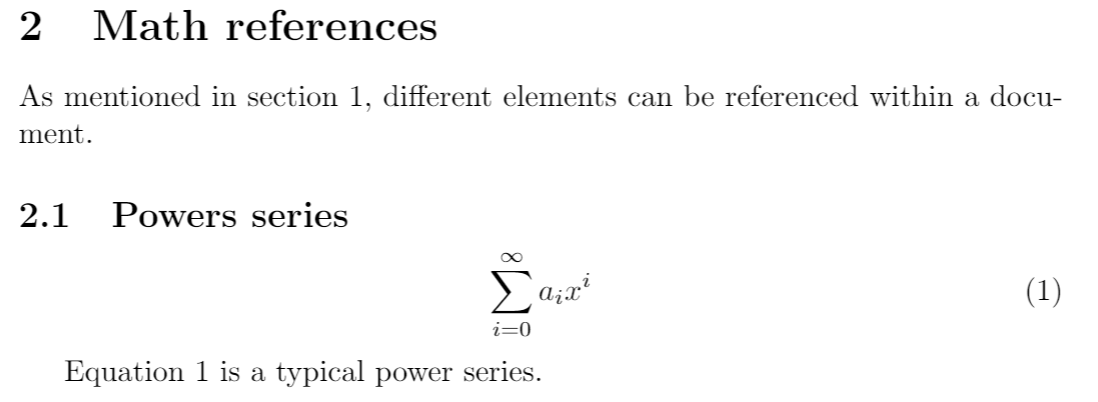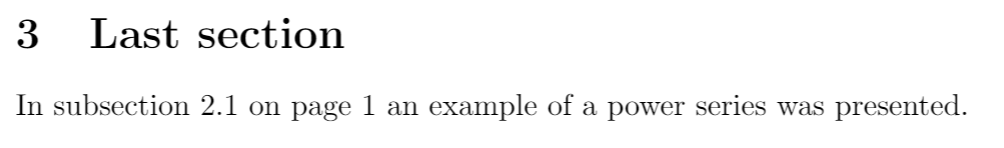Showing first {{hits.length}} results of {{hits_total}} for {{searchQueryText}}{{hits.length}} results for {{searchQueryText}}

If you need to insert cross-references to numbered elements in the document, (like equations, sections and figures) there are commands to automate it in LaTeX. This article explains how. Contents 1 Introduction 2 Referencing sections and chapters 3 Referencing equations, figures and tables 4 Referencing the page of an element 5 Compiling documents with cross references 6 Further reading Introduction Below you can see a simple example of figures cross referenced by their figure caption numbers: \section{Introduction} \label{introduction} This is an introductory paragraph with some dummy text. This section will be referenced later. \begin{figure}[h] \centering \includegraphics[width=0.3\linewidth]{overleaf-logo} \caption{This image will be referenced below.} \label{fig:leaf} \end{figure} You can reference images; for instance, Figure \ref{fig:leaf} shows the \textit{Overleaf} logo.The command \label{ } is used to set an identifier after the caption, that is later used in the command \ref{ } to set the reference.  Open an example in Overleaf Referencing sections and chapters Below an example on how to reference a section \section{Introduction} \label{introduction} This is an introductory paragraph with some dummy text. This section will be referenced later. \begin{figure}[h] \centering \includegraphics[width=0.3\linewidth]{overleaf-logo} \caption{This image will be referenced below.} \label{fig:leaf} \end{figure} You can reference images; for instance, Figure \ref{fig:leaf} shows the \textit{Overleaf} logo. \vspace{0.5cm} \section{Math references} \label{mathrefs} As mentioned in section \ref{introduction}, different elements can be referenced within a document.Again, the commands \label and \ref are used for references. The label is set after the \section statement, i.e. the \label command should be added after the counter number for the section has been generated. This also works on chapters, subsections and subsubsections. See Sections and chapters.  Open an example in Overleaf Referencing equations, figures and tables At the introduction an example of a image referenced was shown, below cross referencing equations is presented. \section{Math references} \label{mathrefs} As mentioned in section \ref{introduction}, different elements can be referenced within a document. \subsection{Powers series} \label{subsection} \begin{equation} \label{eq:1} \sum_{i=0}^{\infty} a_i x^i \end{equation} Equation \ref{eq:1} is a typical power series.For further and more flexible examples with labels and references see Aligning equations with amsmath Tables Inserting Images  Open an example in Overleaf Referencing the page of an element Elements usually are referenced by a number assigned to them, but if you need to, you can insert the page where they appear. \section{Math references} \label{mathrefs} As mentioned in section \ref{introduction}, different elements can be referenced within a document. \subsection{Powers series} \label{subsection} \begin{equation} \label{eq:1} \sum_{i=0}^{\infty} a_i x^i \end{equation} Equation \ref{eq:1} is a typical power series. \vspace{0.5cm} %\Blindtext \section{Last section} In subsection \ref{subsection} on page \pageref{eq:1} an example of a power series was presented.The command \pageref will insert the page where the element whose label is used appears. In the example above the equation 1. This command can be used with all other numbered elements mentioned in this article.  Open an example in Overleaf Compiling documents with cross references On Overleaf cross references work immediately, but for cross references to work properly in your local LaTeX distribution you must compile your document twice. There's also a command that can automatically do the job for all the references to work. For instance, if your document is saved as main.tex. latexmk -pdf main.tex generates the file main.pdf with all cross-references working. To change the output format use -dvi or -ps. Further reading For more information see: Creating a document in LaTeX Mathematical expressions Aligning equations with amsmath Inserting Images Positioning images and tables Indices Glossaries Table of contents Management in a large project Multi-file LaTeX projects Hyperlinks Counters The not so short introduction to LaTeX2ε